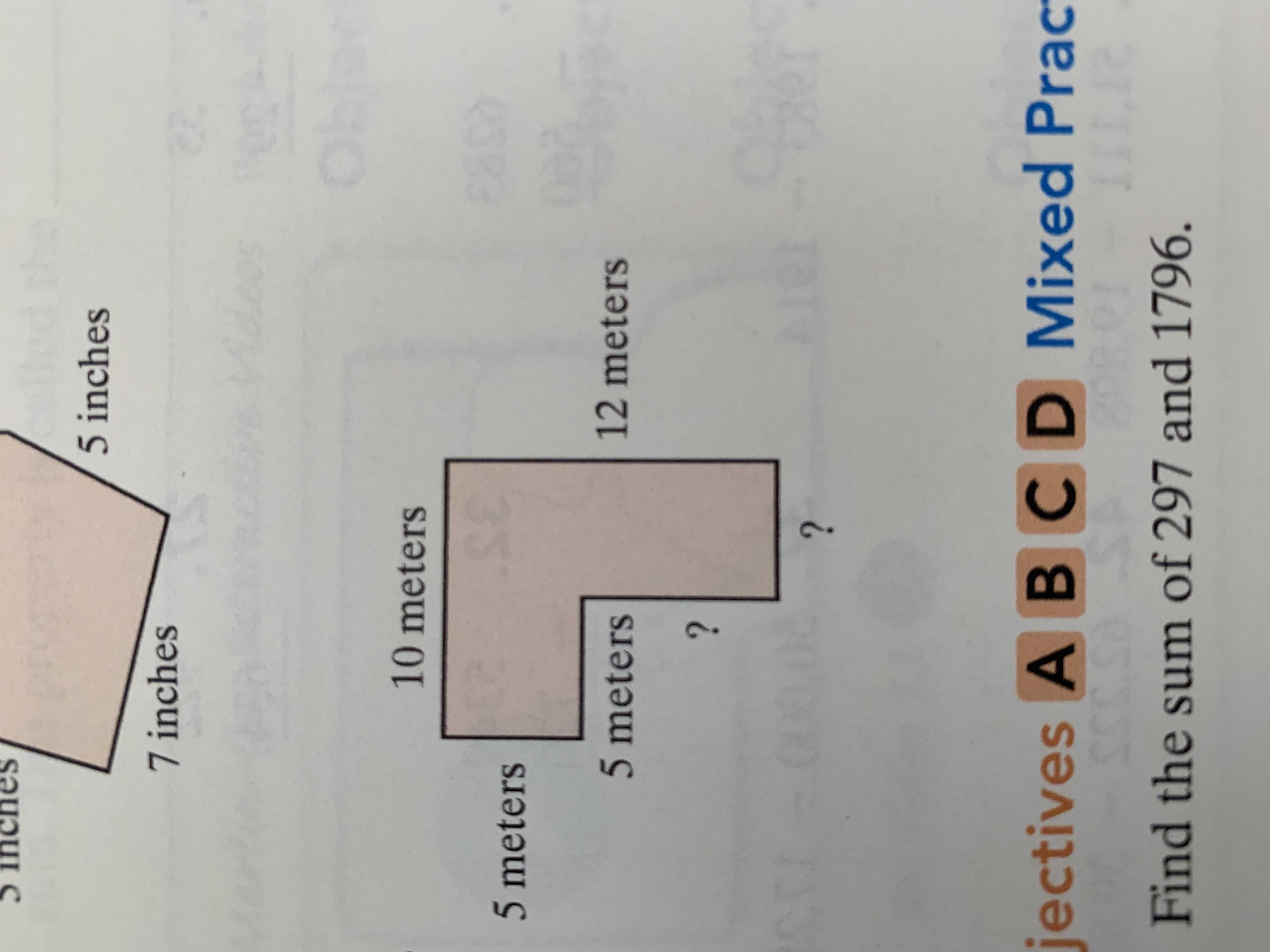# 5 inches7 inches10 meters&95 meters12 meters5 meters??jectives A BCD Mixed Prac2TTFind the sum of 297 and 1796.8

Questionhelp_outlineImage Transcriptionclose5 inches 7 inches 10 meters &9 5 meters 12 meters 5 meters ? ? jectives A BCD Mixed Prac 2TT Find the sum of 297 and 1796. 8 fullscreen
check_circleExpert Solution
Step 1

Lets give name to the figure

In above figure given area is represented by AEFGCDA.

Consider AE extended to B and CG extend to B for ease of our calculation.

Step 2

So now we have two rectangles ABCD and BEFG

Given that

DA = 10 meters , AE = 5 meters , EF = 5 metes . FG = ? and GC = ?

DA = CB = 10 meters   [opposite side of rectangle]

GB = FE = 5 meters    [opposite side of rectangle]

CB = CG + GB = CG + FE&n...

### Want to see the full answer?

See Solution

#### Want to see this answer and more?

Solutions are written by subject experts who are available 24/7. Questions are typically answered within 1 hour*

See Solution
*Response times may vary by subject and question
Tagged in

### Applications of Mathematics